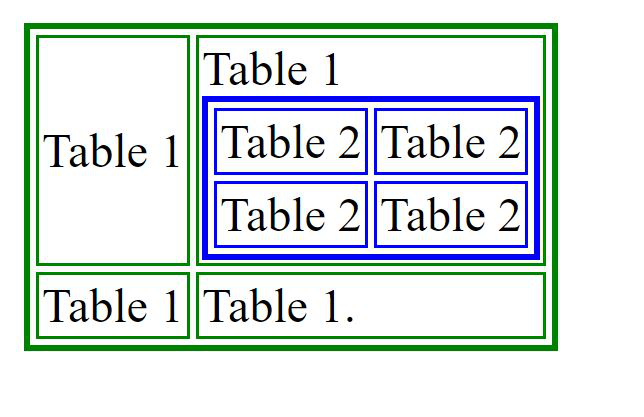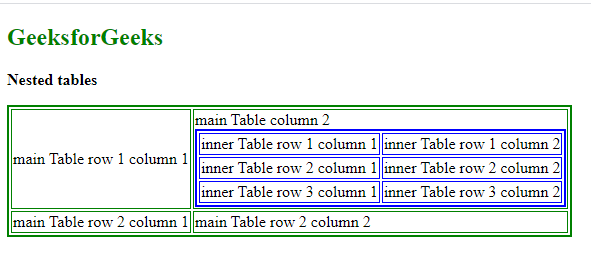Open in App
Not now

# How to create nest tables within tables in HTML ?

• Difficulty Level : Medium
• Last Updated : 14 Aug, 2021

HTML tables are very helpful to structure the content in the form of rows and columns. But sometimes there is a need to add a table within a table. HTML supports this functionality and is known as the nesting of the tables. Tables can be nested together to create a table inside a table.

To create a nested table, we need to create a table using the <table> tag. This table is known as the outer table. The second table that will be nested table is called the inner table. This table is also created using the <table> tag but there is a special thing that must be kept in mind.

Note: The inner table always has to be placed between the <td> ….. </td> of the outer table.

Example 1: Below is an example of creating a nested table. The inner table is added to the second column of the first row of the first table i.e. inside the <td>…</td> tags of the outer table. The tables have been drawn using different colors for better understanding and clarity of the readers. The green border table represents the outer table whereas the inner table has a blue border.

## HTML

 ```<``html``>`` ` `<``body``>``    ``<``table` `border``=``"2"` `bordercolor``=``"green"``>``        ``<``tr``>``            ``<``td``>Table 1``            ``<``td``> Table 1``                ``<``table` `border``=``"2"` `bordercolor``=``"blue"``>``                    ``<``tr``>``                        ``<``td``>Table 2``                        ``<``td``>Table 2``                    ````                    ``<``tr``>``                        ``<``td``> Table 2 ``                        ``<``td``>Table 2``                    ````                ````            ````        ````        ``<``tr``>``            ``<``td``> Table 1 ``            ``<``td``> Table 1. ``        ````    `````` ` ``

Output:Example 2: The above example is modified a little for better understanding.

## HTML

 ```<``html``>`` ` `<``body``>``    ``<``h2` `style``=``"color:green"``>GeeksforGeeks``    ``<``p``><``b``>Nested tables`` ` `    ``<``table` `border``=``"2"` `bordercolor``=``"green"``>``        ``<``tr``>``            ``<``td``>main Table row 1 column 1``            ``<``td``>main Table column 2``                ``<``table` `border``=``"2"` `bordercolor``=``"blue"``>``                    ``<``tr``>``                        ``<``td``>inner Table row 1 column 1``                        ``<``td``>inner Table row 1 column 2``                    ````                    ``<``tr``>``                        ``<``td``>inner Table row 2 column 1 ``                        ``<``td``>inner Table row 2 column 2``                    ````                    ``<``tr``>``                        ``<``td``>inner Table row 3 column 1 ``                        ``<``td``>inner Table row 3 column 2``                    ````                ````            ````        ````        ``<``tr``>``            ``<``td``> main Table row 2 column 1 ``            ``<``td``> main Table row 2 column 2 ``        ````    `````` ` ``

Output:Note: Nested tables can be slow to load, restrictive for layouts, and prevent a more flexible and functional web page. They are lesser recommended from the SEO perspective.

My Personal Notes arrow_drop_up